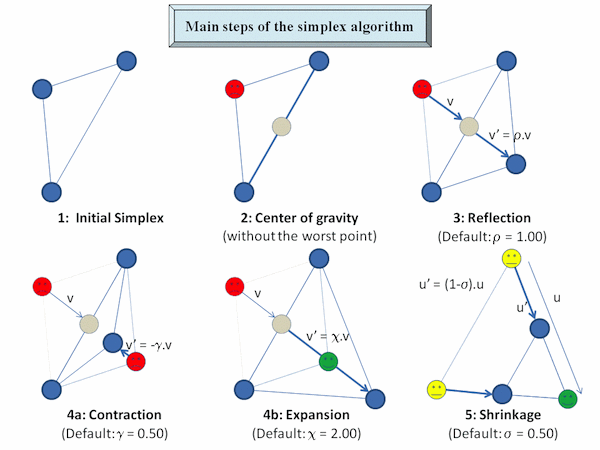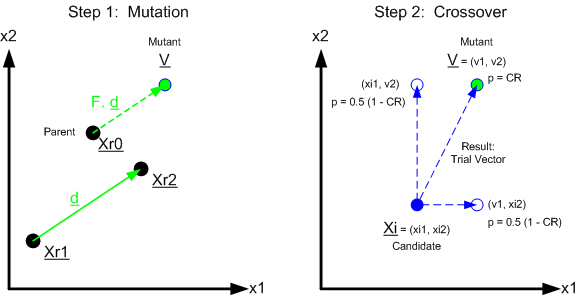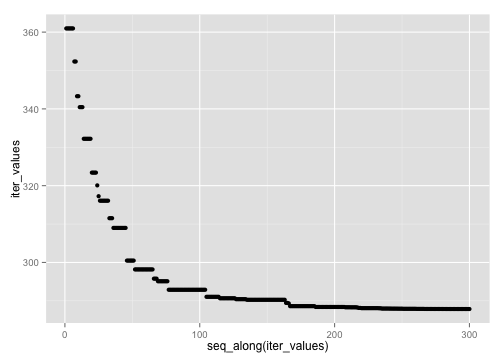# Location Optimization in R

Harlan D. Harris and Alan Briggs

Summary: This document walks through several ways of optimizing locations in R, given ZIP code data about peoples' home and work. Techniques include mapping with the rmaps package (alpha!), continuous optimization with optim, and global optimization with the DEoptim package.

library(knitr)
opts_chunk$set(fig.width = 7, fig.height = 5, echo = TRUE, cache = TRUE, autodep = TRUE) options(stringsAsFactors = FALSE) suppressPackageStartupMessages(library(ggplot2)) # for non-map plots library(zipcode) library(plyr) # for munging data library(compiler) # speed up a few routines a bit suppressPackageStartupMessages(library(DEoptim)) # global optimization suppressMessages(library(rMaps)) # interactive maps! library(munsell) # for colors  ## Setup First, load the ZIP code data, filtering out weird values and such. Then, add ZIP code information, including ZIP centroid latitude and longitude. dat <- subset(read.csv("NYOSP_Locations.csv", colClasses = "character"), select = c(Home.Zip, Work.Zip)) dat <- rename(dat, c(Home.Zip = "home_zip", Work.Zip = "work_zip")) # gr, fix leading zeros dat <- mutate(dat, home_zip = sprintf("%05d", as.numeric(home_zip)), work_zip = sprintf("%05d", as.numeric(work_zip))) data(zipcode) home_zip <- zipcode names(home_zip) <- paste0("home_", names(home_zip)) work_zip <- zipcode names(work_zip) <- paste0("work_", names(work_zip)) dat <- join(dat, home_zip, by = "home_zip") dat <- join(dat, work_zip, by = "work_zip") times_square <- c(40.7577, -73.9857) # lat, long dat <- subset(dat, (abs(home_latitude - times_square[]) < 2 & abs(home_longitude - times_square[]) < 2) | (abs(work_latitude - times_square[]) < 2 & abs(work_longitude - times_square[]) < 2)) dat <- subset(dat, !is.na(home_latitude)) head(dat)  ## home_zip work_zip home_city home_state home_latitude home_longitude ## 1 10016 10018 New York NY 40.75 -73.98 ## 2 10012 10011 New York NY 40.73 -74.00 ## 3 10016 10013 New York NY 40.75 -73.98 ## 4 10027 10038 New York NY 40.81 -73.95 ## 5 10031 10031 New York NY 40.83 -73.95 ## 6 10021 10038 New York NY 40.77 -73.96 ## work_city work_state work_latitude work_longitude ## 1 New York NY 40.76 -73.99 ## 2 New York NY 40.74 -74.00 ## 3 New York NY 40.72 -74.01 ## 4 New York NY 40.71 -74.00 ## 5 New York NY 40.83 -73.95 ## 6 New York NY 40.71 -74.00  nrow(dat)  ##  94  ## 1. Simple Statistics for Locations A simplest approach would be to find modal, mean, and median work locations. # get the most common work zip code simple_mode <- names(which.max(table(dat$work_zip)))
# then look up in the previously-pulled list of work zip codes
simple_mode <- unlist(work_zip[work_zip$work_zip == simple_mode, c("work_latitude", "work_longitude")]) # median's simpler... simple_median <- c(median(dat$work_latitude, na.rm = TRUE), median(dat$work_longitude, na.rm = TRUE)) # trim 10% from each edge of the work locaitons, then mean of that simple_trim_mean <- c(mean(dat$work_latitude, na.rm = TRUE, trim = 0.1), mean(dat$work_longitude, na.rm = TRUE, trim = 0.1)) sum_work_locs <- data.frame(type = c("Modal", "Median", "Trimmed Mean"), lon = c(simple_mode[], simple_median[], simple_trim_mean[]), lat = c(simple_mode[], simple_median[], simple_trim_mean[])) print(sum_work_locs)  ## type lon lat ## 1 Modal -74.00 40.75 ## 2 Median -73.99 40.75 ## 3 Trimmed Mean -73.99 40.75  It would be even more useful to put those locations on a map. ## 2. Mapping Locations Generate functions to work with maps. We're now using the brand-spanking-new rMaps package that lets us draw fancy-pants Leaflet maps! First, plot summary statistic locations on the map. mk_marker <- function(lat, lon, name) { list(type = "Feature", properties = list(name = name, popupContent = name), geometry = list(type = "Point", coordinates = c(lon, lat))) } map_with_markers <- function(latlontype, map, center = times_square, zoomlevel = 11) { map <- Leaflet$new()
map$setView(center, zoom = zoomlevel) map$tileLayer(provider = "Stamen.Toner")
markers <- dlply(latlontype, 1, function(row) with(row, mk_marker(lat, lon,
type)))
names(markers) <- NULL  # so converter creates a list
map$geoJson(markers, onEachFeature = "#! function (feature, layer) {\n layer.bindPopup(feature.properties.popupContent);\n } !#") map } map_with_markers(sum_work_locs)  You're not doing that bad, as it turns out. ## 3. Computing Distances and Costs We've got lats and lons, but we live on a sphere, so we need to write/steal some convenience functions. This is the point-to-point distance from the fossil package, and a vectorized version. # distance in kilometers between two long/lat positions (from 'fossil' # package) earth.dist <- function(long1, lat1, long2, lat2) { rad <- pi/180 a1 <- lat1 * rad a2 <- long1 * rad b1 <- lat2 * rad b2 <- long2 * rad dlon <- b2 - a2 dlat <- b1 - a1 a <- (sin(dlat/2))^2 + cos(a1) * cos(b1) * (sin(dlon/2))^2 c <- 2 * atan2(sqrt(a), sqrt(1 - a)) R <- 6378.145 d <- R * c return(d) } earth.dist.o <- cmpfun(earth.dist) # make it a bit faster  Define a simple point-to-point cost function, then calculate the costs of some recent venues for NYOSP, and plot on a map. # df must have cols work_longitude and work latitude lon and lat must be # scalars scale just scales the units x=2 is cartesian distance p2p_cost <- function(df, lon, lat, scale = 100, x = 2) { sum((earth.dist.o(df$work_longitude, df$work_latitude, lon, lat)/scale)^x, na.rm = TRUE) } # How good or bad are recent locations, for optimizing vs people's work # locations? Recent locations: venues <- data.frame(name = c("pivotal", "aol", "columbia"), lat = c(40.7403372, 40.7308948, 40.8074358), lon = c(-73.9951462, -73.9917096, -73.9625858)) p2p_venue_costs <- ddply(venues, .(name, lat, lon), function(vv) data.frame(cost = p2p_cost(dat, lon = vv$lon, lat = vv$lat))) # print(p2p_venue_costs)  ## name lat lon cost ## 1 aol 40.73 -73.99 327.0 ## 2 columbia 40.81 -73.96 328.7 ## 3 pivotal 40.74 -74.00 326.9  mk_circle <- function(lat, lon, color) { list(type = "Feature", properties = list(color = "black", fillColor = mnsl(sprintf("5PB %d/10", color), fix = TRUE)), geometry = list(type = "Point", coordinates = c(lon, lat))) } plot_with_circles <- function(latloncost, center = times_square, zoomlevel = 10) { map <- Leaflet$new()
map$setView(center, zoom = zoomlevel) map$tileLayer(provider = "Stamen.Toner")

latloncost$cost_color <- with(latloncost, 9 - round(8 * sqrt((cost - min(cost))/(max(cost) - min(cost))))) markers <- dlply(latloncost, 1, function(row) with(row, mk_circle(lat, lon, cost_color))) names(markers) <- NULL # so converter creates a list map$geoJson(markers, pointToLayer = "#! function (feature, latlng) {\n          return L.circleMarker(latlng, {\n      radius: 8,\n      color: feature.properties.color,\n      fillColor: feature.properties.fillColor,\n      weight: 1,\n      opacity: 1,\n      fillOpacity: 0.8\n  });\n      } !#")

map

}

plot_with_circles(p2p_venue_costs, zoom = 11)


The cost function is based on the sums of the point-to-point distances between the target point and each of the work locations:

$\operatorname{cost} = \sum_{\operatorname{workloc}} d(p, \operatorname{workloc})$

where $$d$$ is distance-on-a-sphere, which in this case is extremely close to Euclidean distance.

Technical note: The sum of convex cost functions is provably convex.

To compute distance to a commute, we need to use the “point to line segment” distance calculation, or “point to point” if only one location was provided (unemployed, work at home, classified).


mk_commuteline <- function(lat1, lon1, lat2, lon2) {
if (is.na(lat2)) {
list(type = "Feature", geometry = list(type = "LineString", coordinates = list(c(lon1,
lat1), c(lon1, lat1))))
} else {
list(type = "Feature", geometry = list(type = "LineString", coordinates = list(c(lon1,
lat1), c(lon2, lat2))))
}
}

plot_with_linesegs <- function(lat1, lon1, lat2, lon2, center = times_square,
fn = mk_commuteline) {
map <- Leaflet$new() map$setView(center, zoom = 10)
map$tileLayer(provider = "Stamen.Toner") commute_lines <- llply(seq_along(lat1), function(i) fn(lat1[[i]], lon1[[i]], lat2[[i]], lon2[[i]])) names(commute_lines) <- NULL # so converter creates a list map$geoJson(commute_lines, style = list(color = "#ff5800", weight = 5, opacity = 0.65))

map
}

with(dat, plot_with_linesegs(home_latitude, home_longitude, work_latitude, work_longitude))


Skip the somewhat slow surface-of-a-sphere distance now, and just use a simpler Euclidean distance.

# p-norm computation. p = 2 is cartesian
dist <- function(a, b, c, d, p = 2) {
(abs(a - b)^p + abs(c - d)^p)^(1/p)
}
dist.o <- cmpfun(dist)

# distance from lon/lat to the df with four column names specified in cols
# as (end1_x, end1_y, end2_x, end2_y), where end2 may be missing.  returns a
# vector of costs.
p2ls_cost_v <- function(df, cols, lon, lat, p = 2, km_per_degree = 69.11) {
stopifnot(length(cols) == 4)
stopifnot(all(cols %in% names(df)))
stopifnot(length(lon) == 1)
stopifnot(length(lat) == 1)

# http://stackoverflow.com/questions/849211/shortest-distance-between-a-point-and-a-line-segment

# convert from lat/lon to flat coordinates, using the lat/lon ratio at the
# point in question
param_x = lon * km_per_degree * cos(lat)
param_y = lat * km_per_degree
home_x = df[[cols[]]] * km_per_degree * cos(lat)
home_y = df[[cols[]]] * km_per_degree
work_x = df[[cols[]]] * km_per_degree * cos(lat)
work_y = df[[cols[]]] * km_per_degree

# first, get the home distances
home_dists <- dist.o(home_x, param_x, home_y, param_y, p)

# then, get the distances to the line segment, which may be NA length of the
# segment (squared)
l2 <- (home_x - work_x)^2 + (home_y - work_y)^2
# position of closest point on line
t <- (param_x - home_x) * (work_x - home_x) + (param_y - home_y) * (work_y -
home_y)
t <- t/l2
proj_x <- home_x + t * (work_x - home_x)
proj_y <- home_y + t * (work_y - home_y)
seg_dists <- ifelse(t < 0, home_dists, ifelse(t > 1, dist(work_x, param_x,
work_y, param_y, p), dist(proj_x, param_x, proj_y, param_y, p)))

ifelse(is.na(seg_dists), home_dists, seg_dists)
}
p2ls_cost_v.o <- cmpfun(p2ls_cost_v)

# wrapper function for convenience
p2ls_cost <- function(df, lon, lat, p = 2) {
sum(p2ls_cost_v.o(df, c("home_longitude", "home_latitude", "work_longitude",
"work_latitude"), lon, lat, p))
}


Now, plot the costs of recent venues on a map, using point-to-line-segment.

p2ls_venue_costs <- ddply(venues, .(name, lat, lon), function(vv) data.frame(cost = p2ls_cost(dat,
lon = vv$lon, lat = vv$lat)))

print(p2ls_venue_costs)

##       name   lat    lon  cost
## 1      aol 40.73 -73.99 441.9
## 2 columbia 40.81 -73.96 582.6
## 3  pivotal 40.74 -74.00 430.9

plot_with_circles(p2ls_venue_costs, zoom = 11)


Interestingly, with point-to-line-segment (commute) cost, Pivotal is a lot better than AOL, whereas with point-to-point (work location) cost, Pivotal was only slightly better than AOL. Columbia's not great in either case. Looks the same on the map.

## 4. Mapping Single-Point Costs

Pictures are worth many words. Iterate over a grid to get costs for various locations, then plot.


grd <- expand.grid(lat = seq(from = times_square[] - 0.1, to = times_square[] +
0.1, length.out = 31), lon = seq(from = times_square[] - 0.1, to = times_square[] +
0.1, length.out = 31))
grd <- adply(grd, 1, function(rr) data.frame(cost = p2ls_cost(dat, rr$lon, rr$lat)))
grd$goodness <- 1 - (grd$cost/max(grd$cost)) # grd_json <- # rjson::toJSON(rCharts::toJSONArray2(grd[,c('lat','lon','goodness')], json # = F, names = F))  # TODO: make this better! map <- Leaflet$new()
map$setView(times_square, zoom = 10) map$tileLayer(provider = "Stamen.Toner")

markers <- alply(grd, 1, function(row) with(row, mk_circle(lat[], lon[],
round(goodness[] * 10))))
names(markers) <- NULL  # so converter creates a list
map$geoJson(markers, pointToLayer = "#! function (feature, latlng) {\n return L.circleMarker(latlng, {\n radius: 8,\n color: feature.properties.color,\n fillColor: feature.properties.fillColor,\n weight: 1,\n opacity: 1,\n fillOpacity: 0.8\n});\n } !#") map  The pretty maps show that South of Midtown is the best place for a Meetup. ## 5. Optimizing Single Points Use relatively simple Nelder-Mead optimization to find optimal cost.Is it the global optimum? Seems to be – multiple runs with different initial conditions give the same result. (I've separately run many times, not just the few shown here.) The individual cost functions are not quite convex (all points on the line segment are optima), but it may be that the sum is in practice, at least if you start in a reasonable neighborhood. # wrap for optim() p2ls_cost_opt <- function(params) { p2ls_cost(dat, params[], params[]) } starting_points = alply(venues, 1, function(rr) c(rr$lon, rr$lat)) # call optim, which defaults to Nelder-Mead/Simplex one_loc <- llply(starting_points, function(sp) optim(sp, p2ls_cost_opt, control = list(trace = 0))) print(laply(one_loc, function(ol) ol$par))  # all the same!

##           1     2
## [1,] -73.99 40.75
## [2,] -73.99 40.75
## [3,] -73.99 40.75


single_p2ls_optim = list(x = one_loc[]$par[], y = one_loc[]$par[])

# show the single optim vs the historical venues
map <- plot_with_circles(p2ls_venue_costs, zoom = 12)
map$marker(c(single_p2ls_optim$y, single_p2ls_optim$x), bindPopup = "optimal") map  30th St between 5th and 6th! What's there?## 6. Computing N-Point Costs If there are multiple locations, define the cost to be the minimum cost, for each person, to get to any location from their commute. $\operatorname{cost} = \sum_{\operatorname{person}} min_{\operatorname{loc}_i} d(\operatorname{loc}_i, \operatorname{commute}_{\operatorname{person}})$ colnames <- c("home_longitude", "home_latitude", "work_longitude", "work_latitude") p2lsN_cost <- function(dat, latlons, p = 2) { # for each pair of latlons, calc p2ls_cost costs <- laply(1:(length(latlons)/2), function(i) p2ls_cost_v.o(dat, colnames, latlons[[i * 2 - 1]], latlons[[i * 2]], p)) sum(aaply(costs, 2, min)) } p2lsN_cost.o <- cmpfun(p2lsN_cost) p2lsN_cost_opt <- function(latlons) { p2lsN_cost.o(dat, latlons) } # get cost for two example triples of locations venue_triple <- unlist(dlply(venues, 1, function(rr) c(rr$lon, rr$lat))) tri1_cost <- p2lsN_cost_opt(venue_triple) tri2_cost <- p2lsN_cost_opt(unlist(c(venue_triple[1:4], single_p2ls_optim$y,
single_p2ls_optim$x)))  mk_polygon <- function(lats, lons, color = "black") { stopifnot(length(lats) == length(lons)) coord_list <- llply(seq_along(lats), function(i) c(lons[[i]], lats[[i]])) list(type = "Feature", properties = list(name = "mypolygon", color = color), geometry = list(type = "Polygon", coordinates = list(coord_list))) } plot_with_triangles <- function(listoflists, center = times_square, zoomlevel = 11, style = "#! false !#") { map <- Leaflet$new()
map$setView(center, zoom = zoomlevel) map$tileLayer(provider = "Stamen.Toner")

polygons <- llply(listoflists, function(l) mk_polygon(l$lat, l$lon, l$color)) map$geoJson(polygons, style = style)

map
}

venues_new <- subset(venues, name != "columbia")
venues_new <- rbind(venues_new, data.frame(name = "optimal", lat = single_p2ls_optim$y, lon = single_p2ls_optim$x))
plot_with_triangles(list(list(lat = venues$lat, lon = venues$lon, color = "blue"),
list(lat = venues_new$lat, lon = venues_new$lon, color = "red")), style = "#!  function(feature) {\n                        return { color: feature.properties.color }\n                     } !#")


The three past locations are substantially better in the 3-location cost function (337.6129, blue) than the 1-location optimum plus two other downtown locations (350.8927, red)! Having Columbia in the mix definitely makes attendance easier for some people.

## 7. Local Minima in N-Point Optimization

We want to use the new 3-point-to-line-segment cost function to find 3 optimal locations. Now, I'm using the “L-BFGS-B” algorithm, which is an iterative quasi-Newton algorithm that supports bounds on the parameters.

# constrain the points to be not too far from DC
lower_box <- rep(c(times_square[] - 0.5, times_square[] - 0.5), 3)
upper_box <- rep(c(times_square[] + 0.5, times_square[] + 0.5), 3)

# this takes a few minutes ... But only need a few runs to see the issue.
set.seed(1)
nruns = 5

starting_points = rlply(nruns, function(i) venue_triple + rnorm(6, sd = 0.2))
three_loc <- llply(starting_points, function(sp) optim(sp, cmpfun(p2lsN_cost_opt),
method = "L-BFGS-B", lower = lower_box, upper = upper_box, control = list(trace = 1)))

## iter   10 value 355.450921
## iter   20 value 354.640800
## final  value 354.359662
## converged
## iter   10 value 304.504263
## iter   20 value 300.814285
## iter   30 value 299.244100
## final  value 299.243890
## converged
## iter   10 value 366.796349
## iter   20 value 322.362071
## final  value 309.318992
## stopped after 26 iterations
## iter   10 value 332.251092
## iter   20 value 322.328787
## iter   30 value 320.912275
## final  value 320.912174
## converged
## iter   10 value 317.233032
## iter   20 value 292.837203
## final  value 291.014566
## stopped after 22 iterations


I'll refer you to Wikipedia's entry on BFGS for a technical overview. Roughly (as I understand it):

1. At any given point, you can measure the local curvature of the surface.
2. Based on your current estimate of the curvature of the surface, use trial-and-error to find a good distance to go in the seemingly-best direction.
3. Update your estimate of the curvature of the surface based on how far you moved and how the local curvature changed.

L-BFGS is memory-efficient in high-dimensional spaces, at the cost of some precision. L-BFGS-B adds low/high constraints on each dimension.

To see if there are local minima, run a few times with different starting points. Plot the triangles found in each run on top of each other. Uh oh.

three_loc_pars <- laply(three_loc, function(ol) ol$par) print(three_loc_pars)  ## aol1 aol2 columbia1 columbia2 pivotal1 pivotal2 ## [1,] -73.97 40.69 -73.99 40.89 -73.99 40.75 ## [2,] -73.99 40.74 -73.96 40.81 -73.76 40.77 ## [3,] -74.38 40.27 -73.99 40.74 -73.95 40.81 ## [4,] -73.99 40.75 -74.11 40.97 -74.37 40.58 ## [5,] -73.95 40.81 -74.49 40.61 -73.99 40.74   # turn these into triangles and plot them df3_to_list <- function(dd, color = "black") { ret <- alply(dd, 1, function(row) list(lat = as.vector(row[c(2, 4, 6)]), lon = as.vector(row[c(1, 3, 5)]), color = color)) names(ret) <- NULL ret }  plot_with_triangles(df3_to_list(three_loc_pars), style = "#! function(feature) {\n return { color: feature.properties.color }\n } !#", zoomlevel = 9)  It found widely differing 3-location “solutions” with each initial starting point. Not good. Let's throw some horsepower at this to be more confident we can find a global solution. ## 8. Global N-Point Optimization Use Differential Evolution, an algorithm that uses quasi-Darwinian evolution of populations of candidate solutions, with Nelder-Mead-like gradient heuristics at each generation, to (likely) find the global optimum. # Could use the historical points as starting points, but it turns out not # to be a good enough location to matter much, so letting the algorithm pick # random points in the bounding box instead. # This takes a few minutes. NP should be at least 60, itermax seems to # converge after a few hundred, and strategy doesn't seem to make much # difference for this decision surface. three_loc_de <- DEoptim(cmpfun(p2lsN_cost_opt), lower = lower_box, upper = upper_box, control = list(trace = FALSE, NP = 100, itermax = 300, strategy = 3)) # get a copy of coffee... Note that not using parallel because running on a # Mac in an R console...  Here's a nice illustration of the simplest version of the algorithm, from a blog post.## 9. Mapping N-Point Solutions Show the DE convergence, and the global optimum on maps. # DEoptim bug makes plot.DEoptim not work right -- do it by hand iter_values <- three_loc_de$member$bestvalit[1:three_loc_de$optim$iter] qplot(seq_along(iter_values), iter_values)plot_with_triangles(df3_to_list(data.frame(t(three_loc_de$optim\$bestmem)), color = "green"),
style = "#!  function(feature) {\n                        return { color: feature.properties.color }\n                     } !#",
zoomlevel = 11)


And we're done with the demo!

## 10. Possible Fun Extensions

In roughly easiest-to-hardest order.

• Change the cost function to use Manhattan distance (p=1) instead of Euclidean distance (p=2). Find the single-location optimum location.

• Change the cost function (p2ls_cost_opt) to be the mean of the two best point-to-line-segment costs, instead of just the single best. How does this change the best 3-location optimum?

• Try another global optimization method, such as simulated annealing.

• Profile this R code, find out why it's slow, and fix it. Supporting multicore would be low-hanging fruit.

• Is there a variant of the cost function in the 3-location problem that's convex, allowing faster optimization?